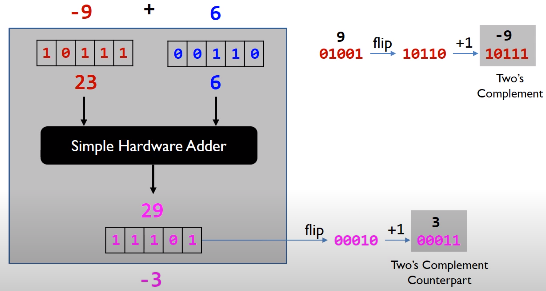In this article, we will learn how to use Bitwise operators and some tricks to deal with our common problems. Let’s get started.

## Introduction to Bitwise operators

Bitwise operators are operators that perform with each bit of a number. But we can use them with some integral types such as char, short, int, …

There are some operators:

• Bitwise AND `&`
• Bitwise OR `|`
• Bitwise XOR `^`
• Bitwise Complement `~`
• Shift operator `>>` or `<<`
1. Bitwise AND `&`

`````` 1 & 1 = 1
1 & 0 = 0
0 & 0 = 0
0 & 1 = 0
``````

So if both bits are 1, then AND operator gives 1, otherwise 0.

2. Bitwise OR `|`

`````` 1 | 1 = 1
1 | 0 = 1
0 | 1 = 1
0 | 0 = 0
``````

If either of bits are 1, then OR operator gives 1, otherwise 0.

3. Bitwise XOR `^`

`````` 1 ^ 1 = 0
1 ^ 0 = 1
0 ^ 1 = 1
0 ^ 0 = 0
``````

When performing XOR on two integers, the XOR operation is calculated on each pair of bits (the two bits at the same index in each number).

`````` 5 ^ 6  // gives 3

// At the bit level:
//     0101  (5)
//   ^ 0110  (6)
//   = 0011  (3)
``````
4. Bitwise Complement `~`

`````` ~5 = ~0101 = 1010
``````

This operator will return the one’s complement representation of the input value by inversing all bits in a variable, 1 to 0, or 0 to 1.

Then the highest bit is 1, the complier or OS will give the two’s complement for this number. It looks like that the below image.

`````` ~5 = 1010 (binary) = 10 (decimal)  // (the one's complement)
-6 = ~0110 = 1001 --> (+1) --> 1010
``````

The two’s complement simplifies the hardware implementation of arithmetic functions.

• For addition or substraction in two’s complement, the hardware can ignore the sign of operands if signed integers are represented.
• For multiplication, the multiplication hardware can also ignore the sign of operands if the product is required to keep the same number of bits as operands.

–> This property simplifies the hardware design for addition, substraction, and multiplication. This property does not hold true for division.5. Shift operator

A bit shift moves each digit in a number’s binary representation left or right. There are three main types of shifts:

• Left shifts

When shifting left, the most-significant bit is lost, and a 0 bit is inserted on the other end.

The left shift operator is usually written as “«”.

``````  0010 << 1  →  0100
0010 << 2  →  1000
``````

A single left shift multiplies a binary number by 2:

``````  0010 << 1  →  0100

0010 is 2
0100 is 4
``````
• Logical Right shifts

When shifting right with a logical right shift, the least-significant bit is lost and a 0 is inserted on the other end.

``````  1011 >>> 1  →  0101
1011 >>> 3  →  0001
``````

For positive numbers, a single logical right shift divides a number by 2, throwing out any remainders.

``````  0101 >>> 1  →  0010

0101 is 5
0010 is 2
``````
• Arithmetic Right shifts

When shifting right with an arithmetic right shift, the least-significant bit is lost and the most-significant bit is copied.

Languages handle arithmetic and logical right shifting in different ways. Java provides two right shift operators: » does an arithmetic right shift and »> does a logical right shift.

``````  1011 >> 1  →  1101
1011 >> 3  →  1111

0011 >> 1  →  0001
0011 >> 2  →  0000
``````

The first two numbers had a 11 as the most significant bit, so more 11’s were inserted during the shift. The last two numbers had a 0 as the most significant bit, so the shift inserted more 0’s.

If a number is encoded using two’s complement, then an arithmetic right shift preserves the number’s sign, while a logical right shift makes the number positive.

``````  // Arithmetic shift
1011 >> 1  →  1101
1011 is -5
1101 is -3

// Logical shift
1111 >>> 1  →  0111
1111 is -1
0111 is  7
``````

## When to use

• when performing update and query operations of Binary Indexed Tree.

## Benefits and Drawbacks

1. Benefits

• fast.
2. Drawbacks

• It’s difficult to understand, read code, maintain code.

## Some tricks in Bit Manipulation

1. Clear all bits from LSB to ith bit

`````` mask = ~((1 << (i + 1) - 1);
``````
2. Clear all bits from MSB to ith bit

`````` mask = (1 << i) - 1;
``````
3. Check whether a character is upper case

`````` char ch = 'A';
boolean isUpperCase = (ch & 32) == 0;
``````

In ASCII, the distance between upper cases and lower cases is 32. So, if a character is upper case, it does not contain 5th bit –> 0010 0000.

4. Convert upper case to lower case

`````` char ch = 'A';
char lowerCase = ch | 32;
``````
5. Convert lower case to upper case

`````` int distance = 32;
char ch = 'a';
char upperCase = ch & ~distance; // we get all characters that excepts 5th bit.
``````

## Wrapping up

• Understanding the meaning of all basic bitwise operators.

Refer:

https://www.geeksforgeeks.org/bits-manipulation-important-tactics/

https://www.vojtechruzicka.com/bit-manipulation-java-bitwise-bit-shift-operations/

https://www.log2base2.com/C/bitwise/bitwise-ones-complement-operator-in-c.html

https://www.interviewcake.com/concept/java/bit-shift